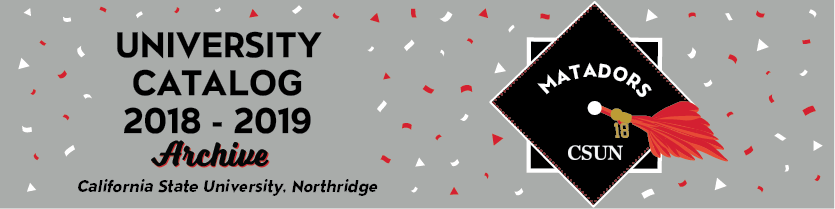## This is an archive of the 2018-2019 University Catalog. To access the most recent version, Please visit catalog.csun.edu.## Program Description

Mathematicians today are engaged in a wide variety of activities. Research mathematicians create new theories and techniques. Applied mathematicians use that theory and mathematical modeling to solve problems in economics, science, medicine, engineering and management. Teachers of mathematics develop new ways to teach mathematical concepts to children and adults. University-level mathematics involves more than algorithms and computational techniques. Mathematics majors also learn to construct proofs and how to approach a real-life problem from a mathematical point of view.

### Double Major

A student pursuing either a B.A. or a B.S. degree may combine a second major with Mathematics. In this circumstance, upon approval of an advisor, 6 units of upper division electives may be satisfied by courses in the second major. The remaining electives must be taken in the Department of Mathematics. Under certain rare conditions, the physics requirement in the lower division core may be replaced by appropriate coursework in the second major. Prior approval must be obtained from an advisor and the department chair for this latter occurrence.

## Program Requirements

### Requirements

In addition to University residency requirements for a bachelor’s degree, the student must complete a minimum of 18 units of upper division Mathematics in residence at CSUN with the approval of a Mathematics advisor. Students in B.A. degree programs must fulfill the University requirement of at least 40 units of upper division coursework overall.

It is assumed that the student has a facility in mathematics normally gained by recent completion of four years of high school mathematics through trigonometry and “Mathematical Analysis.” Because of the variation in curricula at the high school level, it is necessary to obtain satisfactory scores on the Mathematics Placement Test (MPT) and Entry Level Mathematics Exam (ELM)* to enter the first mathematics course in the program, MATH 150A. Without satisfactory scores, a student will need to complete additional coursework.

*Effective Fall 2018, the ELM Exam has been replaced with Multiple Measures Assessment.

#### 1. Lower Division Core for All Programs (23-24 units)

The student must complete the lower division core and one of the Mathematics options, and he or she must have at least a 2.0 GPA for all upper division units required in the major.

#### 3. Upper Division Electives (9 units)

Upper division Mathematics electives selected with advisor approval, not to include MATH 310/L, 311, 312, 331, 391 or 490. Students considering graduate work in mathematics are strongly advised to include MATH 450B, 455 and 460 in their program of study or to complete a B.S. degree in Mathematics.

#### 4. General Education (48 units)

Undergraduate students must complete 48 units of General Education as described in this Catalog.
Basic Skills Mathematics and Lifelong Learning are satisfied by required courses in the major. PHYS 220A/AL partially satisfies the Natural Sciences section. Students are encouraged to take PHIL 230 to satisfy the Basic Skills Critical Thinking requirement.

## Contact

Department of Mathematics
Chair: Rabia Djellouli
Live Oak Hall (LO) 1300
(818) 677-2721

## Student Learning Outcomes

Students shall be able to:

1. Devise proofs of basic results concerning sets and number systems.
2. Rigorously establish fundamental analytic properties and results such as limits, continuity, differentiability and integrability.
3. Demonstrate facility with the objects, terminology, and concepts of linear algebra.
4. Demonstrate facility with the terminology, use of symbols, and concepts of probability.
5. Write simple computer programs to perform computations arising in the mathematical sciences.